# The Open Construction & Building Technology Journal

ISSN: 1874-8368 ― Volume 15, 2021
RESEARCH ARTICLE

# Risk-Based Decision Making Method for Selecting Slope Stabilization System in an Abandoned Open-Pit Mine

Mahnoush Gharehdaghi1, Hesam S. Tehrani1, Ali Fakher2, *
1 School of Civil Engineering, College of Engineering, University of Tehran, Tehran, Iran
2 Department of Civil Engineering, University of Tehran, Tehran, Iran

## Abstract

### Background:

The construction and stabilization of deep excavations are associated with several uncertainties due to heterogeneous geological conditions. Therefore, the conventional methods of slope stability analysis do not provide reasonable results.

### Aim:

Hence, it is logical to perform reliability analysis and also risk assessment to make a wiser decision under uncertainty for choosing the proper stabilization method of slopes.

### Methods:

In this regard, a real case study, a 50-meter-deep abandoned open-pit mine, is considered. In the past, the studied deep excavation was located in a rural area, away from the important structures. However, due to the development of the city, the open-pit mine is now located in the city. Furthermore, the Kan River is located on the eastern side of the excavation. Deterministic analysis showed that that Factor of Safety is not sufficient for permanent condition; thus, the deep excavation may have destructive impacts on the adjacent structures and infrastructures by putting them in danger in the case of failure.

### Results:

These circumstances resulted in using reliability analysis and risk assessment using non-deterministic approach. Random Set Finite Element Method (RS-FEM), a non-probabilistic method, is used in determining how much the slope is reliable. The upper and lower bounds of probability of excessive displacement and probability of failure are obtained using RS-FEM by Plaxis2D software. Afterward, HAZUS is successfully used to quantify the economic risk of different stabilization alternatives by defining various scenarios in order to consider the consequences of excavation failure on adjacent utilities and infrastructures.

### Conclusion:

The best alternative is defined as the stabilization method with the lowest economic risk. As a result, it is noticeable that this paper provides a comprehensive methodology for decision making, based on reliability analysis and risk assessment, in stabilizing slopes.

Keywords : Deep excavation, Open-pit mine, Slope stability, Reliability analysis, Random set, Finite element, Risk assessment.

### Article Information

#### Identifiers and Pagination:

Year: 2020
Volume: 14
Issue: Suppl-1, M2
First Page: 198
Last Page: 217
Publisher Id: TOBCTJ-14-198
DOI: 10.2174/1874836802014010198

#### Article History:

Acceptance Date: 15/01/2020
Electronic publication date: 24/08/2020
Collection year: 2020

open-access license: This is an open access article distributed under the terms of the Creative Commons Attribution 4.0 International Public License (CC-BY 4.0), a copy of which is available at: (https://creativecommons.org/licenses/by/4.0/legalcode). This license permits unrestricted use, distribution, and reproduction in any medium, provided the original author and source are credited.

* Address correspondence to this author at Department of Civil Engineering, University of Tehran, Tehran, Iran; Tel: +98 9123380360; E-mail: Afakher@ut.ac.ir

## 1. INTRODUCTION

Nowadays, the rate of underground construction, such as deep excavation and tunneling, has increased. In the case of deep excavation, this rate changes very quickly. It can be attributed to the growth of the population and lack of adequate land in urban areas to develop cities in order to settle people, develop malls and parking, or extraction of ground soils. The mining process is conducted to get mineral and soil components for diverse uses, including agriculture and construction [1S. Mwangi, "Management of river systems in east Africa", Nairobi, Macmillan, .]. It takes years for sand and gravel to be formed as a result of erosion of mountain rocks, but they can be extracted in a matter of days [2M. Tariro, Case studies of environmental impacts of sand mining and gravel extraction for urban development in Gaborone, MSc dissertation thesis, University of South Africa, .]. The mining of sand and gravel can be performed on open areas, beaches, inland dunes, and mountainsides. Unscientific mining contributed to severe problems around mining areas [3N.M. Saviour, "Environmental impacts of soil and sand mining: A Review", Int. J. Sci. Environ. Technol, vol. 1, no. 3, pp. 125-134.]. Apparently, there are no strict rules to regulate soil extraction. Hence, deep and wide pits are abandoned after the collection of sand and gravel [4K. Mbaiwa, A Handbook of socio economic and environmental impacts of mining and development, Macmillan: Gaborone, .]. Such activity leads to detrimental effects on the ecosystem, and it may put the health of people in danger due to the possible instability of the deep excavation. There are for operations used for mining sand and gravel from open-pit mines in the USA, including site clearing to remove vegetation, mining, processing, and eventually reclamation of the mined area [5R. Schaetzl, "Sand and gravel mining for aggregate", https://www.msu.edu/-soils]. Therefore, the improvement of the excavated area is necessary to prevent the negative impacts of pits of sand and gravel, which are abandoned after used. One of the crucial activities in this regard is stabilizing the slopes of the deep excavation to make sure it would not threaten the surrounding area.

There are different methods to analyze deep excavation stabilization they can be divided into deterministic and non-deterministic approaches, respectively. Uncertainties in the determination of the in situ soil parameters for each soil layer force researchers to tend to the non-deterministic approaches. Therefore, deterministic analysis method cannot be appropriate sources of judging the stability of the excavation. Accordingly, the tendency to the non-deterministic analysis method is increased. It can be expected that theories and numerical models used in risk analyses play an important role in the near future [6A. Nasekhian, and H.F. Schweiger, "Random set finite element method application to tunneling", Int. J. Reliab. Safety, vol. 5, no. 3-4, pp. 299-319.
[http://dx.doi.org/10.1504/IJRS.2011.041182]
]. Input data used in numerical modeling usually are inadequate; therefore, the probability density function cannot be determined precisely. Hence, this problem made researchers propose relatively imprecise methods to solve the problem. Methods such as Fuzzy Set theory [7L.A. Zadeh, "Fuzzy sets", Inf. Control, vol. 8, pp. 338-353.
[http://dx.doi.org/10.1016/S0019-9958(65)90241-X]
-10P.H. Chuang, "Use of fuzzy sets for evaluating shear strength of soils", Comput. Geotech., vol. 17, pp. 425-446.
[http://dx.doi.org/10.1016/0266-352X(95)94914-C]
], Convex Model [11Y. Ben-Haim, and I. Elishakoff, Convex Models of Uncertainty in Applied Mechanics., 1st edElsevier: Amsterdam, .], Evidence theory [12A.P. Dempster, "Upper and lower probabilities induced by a multivalued mapping", Ann. Math. Stat., pp. 325-339.
[http://dx.doi.org/10.1214/aoms/1177698950]
-14L.C. Pereira, L.J.N. Guimarães, B. Horowitz, and M. Sánchez, "Coupled hydro-mechanical fault reactivation analysis incorporating evidence theory for uncertainty quantification", Comput. Geotech., vol. 56, pp. 202-215.
[http://dx.doi.org/10.1016/j.compgeo.2013.12.007]
], Random Set theory [15D.G. Kendall, Foundations of a theory of random sets., Wiley: New York, .-19H. Shen, and S.M. Abbas, "Rock slope reliability analysis based on distinct element method and random set theory", Int. J. Rock Mech. Min. Sci., vol. 61, pp. 15-22.
[http://dx.doi.org/10.1016/j.ijrmms.2013.02.003]
], Interval analysis [20R.E. Moore, "Interval analysis", -22H. Yang, Y. Li, and Y. Xue, "Interval uncertainty analysis of elastic bi-modular truss structures", Inverse Probl. Sci. Eng., vol. 23, pp. 578-589.], Random Field [23D. Chen, D. Xu, G. Ren, Q. Jiang, G. Liu, L. Wan, and N. Li, "Simulation of cross-correlated non-Gaussian random fields for layered rock mass mechanical parameters", Comput. Geotech., vol. 112, pp. 104-119.
[http://dx.doi.org/10.1016/j.compgeo.2019.04.012]
-27Y.J. Li, M.A. Hicks, and P.J. Vardon, "Uncertainty reduction and sampling efficiency in slope designs using 3D conditional random fields", Comput. Geotech., vol. 79, pp. 159-172.
[http://dx.doi.org/10.1016/j.compgeo.2016.05.027]
], Possibility theory [28J. Rohmer, and C. Baudrit, "The use of the possibility theory to investigate the epistemic uncertainties within scenario-based earthquake risk assessments", Nat. Hazards, vol. 56, pp. 613-632.
[http://dx.doi.org/10.1007/s11069-010-9578-6]
], and Imprecise Probabilities [29P. Walley, Statistical reasoning with imprecise probabilities., Chapman and Hall: London, .
[http://dx.doi.org/10.1007/978-1-4899-3472-7]
] has been prepared.

Among the aforementioned methods, Random Set theory because of its efficiency and simplicity is one of the best methods for reliability analyses. Several authors have provided the Random Set theory as an appropriate mathematical model to deal with uncertainty dominating some of the shortcomings of the “classical” probability theory. Tonon et al. [30F. Tonon, A. Mammino, and A. Bernardini, "A random set approach to the uncertainties in rock engineering and tunnel lining design", Proceedings of ISRM International Symposium on Prediction and Performance in Rock Mechanics and Rock Engineering (EUROCK ’96, vol. 2, pp. 861-868.-32F. Tonon, A. Bernardini, and A. Mammino, "Reliability analysis of rock mass response by means of Random Set Theory", Reliab. Eng. Syst. Saf., vol. 70, no. 3, pp. 263-282. [b].
[http://dx.doi.org/10.1016/S0951-8320(00)00059-4]
] illustrated the application of Random Set Theory in rock mechanics and performed a reliability analysis of a tunnel lining by using Random Set Theory. Thereupon, Peschl [33G.M. Peschl, Reliability analysis in geotechnics with the random set element method, Dissertation, Graz University of Technology, .], Schweiger and Peschl [34H.F. Schweiger, and G.M. Peschl, "Basic concepts and applications of random sets in geotechnical engineering", Book Series CISM International Centre for Mechanical Sciences, vol. 491, pp. 113-126.
[http://dx.doi.org/10.1007/978-3-211-73366-0_4]
] have developed Random Set Theory in the field of finite element method that is called Random-Set-Finite-Element-Method (RS-FEM). RS-FEM is an efficient and applicable framework for performing reliability analyses in geotechnical engineering problems. For additional information about fundamental concepts of Random Set theory and RS-FEM process, readers can refer to the work of Tonon & Mammino [30F. Tonon, A. Mammino, and A. Bernardini, "A random set approach to the uncertainties in rock engineering and tunnel lining design", Proceedings of ISRM International Symposium on Prediction and Performance in Rock Mechanics and Rock Engineering (EUROCK ’96, vol. 2, pp. 861-868.-33G.M. Peschl, Reliability analysis in geotechnics with the random set element method, Dissertation, Graz University of Technology, .], Schweiger & Peschl [34H.F. Schweiger, and G.M. Peschl, "Basic concepts and applications of random sets in geotechnical engineering", Book Series CISM International Centre for Mechanical Sciences, vol. 491, pp. 113-126.
[http://dx.doi.org/10.1007/978-3-211-73366-0_4]
, 35G.M. Peschl Schweiger, "Reliability analysis in geotechnics with the random set finite element method", Comput. Geotech., vol. 32, no. 6, pp. 422-435.
[http://dx.doi.org/10.1016/j.compgeo.2005.07.002]
]. The theory of random sets provides a general framework for coping with set-based data and discrete probability distributions. When ranges of input data are only used, the analysis gives similar results as Interval Analysis, and when numerous data are available, the results are as same as Monte-Carlo simulation [34H.F. Schweiger, and G.M. Peschl, "Basic concepts and applications of random sets in geotechnical engineering", Book Series CISM International Centre for Mechanical Sciences, vol. 491, pp. 113-126.
[http://dx.doi.org/10.1007/978-3-211-73366-0_4]
]. Accordingly, the RS-FEM has been chosen as an appropriate framework to apply reliability analysis in deep excavation projects in this paper.

Decision making analysis as a framework for dealing with difficult decision processes is an analytical technique. This method provides a structured procedure to help a decision maker to think systematically about complicated problems in order to improve the quality of resulting decision [36R. T., Clemen, Making Hard Decision., 2nd edDuxbury Press: United States of America, .]. Kong [37W.K. Kong, "Risk assessment of slopes", Q. J. Eng. Geol. Hydrogeol., vol. 35, no. 3, pp. 213-222.
[http://dx.doi.org/10.1144/1470-9236/2000-39]
] performed the risk analysis of the probability of fatality occurrence (or probability of loss of life) resulting from natural landslide or rock fall.

This article aims to provide a thorough risk assessment process for selecting the best design alternative for risk-reducing purposes. The uncertainty and variability in soil parameters emphasized the process of quantification and qualifying likelihoods and consequence. In addition, this paper also aims to provide a framework to quantify geotechnical risk in slopes such as an abandoned open-pit mine. Characterizing various types of uncertainties related to geotechnical design properties and calculation models is of great importance. Hence, risk management, the work of identification, evaluation and ranking of risks in a project, are essential. The procedure is exemplified with the case study of a deep excavation, which is a part of large, abandoned open-pit mine of sand and gravel. Recently, it is decided to stabilize the slopes in order to decrease the probability of failure and prevent negative consequences. Moreover, some architectural plans are suggested to use the land around it by developing green spaces and constructions. In this paper, the best design alternative for stabilization of the steep slopes is chosen according to the existing and future conditions of the problem of interest. The best stabilization method should provide enough space for the execution of future architectural plans and has the lowest economic risk.

## 2. MATERIALS AND METHODS

### 2.1. Case Study

Pits of mines of sand and gravel are located in the west of Tehran, the capital city of Iran. Some of these mines are excavated to a great depth unscientifically. The plan area of the project is approximately 10000 m2, and the depth of the excavation varies from 39 m to 50 m. Additionally, the steepness of the slopes is variable. In order to opt for the initial stabilization method, the most critical slope (1 H: 2.8 V) and the depth of 50 m was chosen as the most critical slope. This mine was initially far from the city. Hence, it did not put the equipment and structures in jeopardy of destruction. However, due to the development of the urban area, these pits are now adjacent to highways and residential areas, as shown in Fig. (1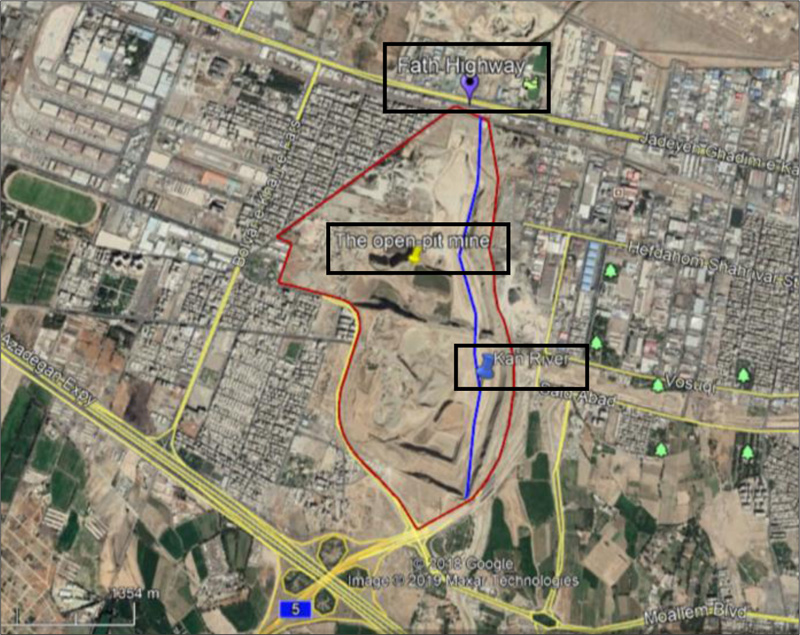). Fig. (1) also shows the aerial picture of the studied excavation and the neighboring area. The northern side is adjacent to the Fath highway. The western and southern sides of the project are contiguous to high-rise buildings far from the Ferbet excavation. Therefore, the instability of the slopes threatens the nearby structures and infrastructure. A part of the excavated area is shown in Fig. (2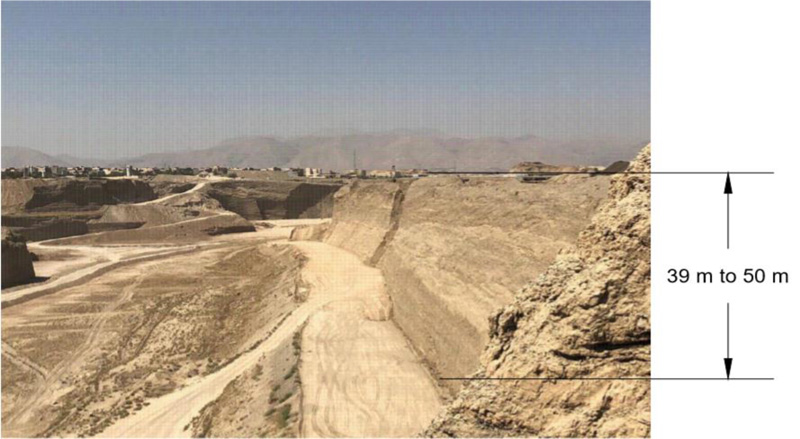).

In addition, the presence of the Kan River, which is shown in Fig. (3), on the eastern side of the project exacerbates the situation and heightens the risk of destruction because of heavy rainfall and flood. Fig. (4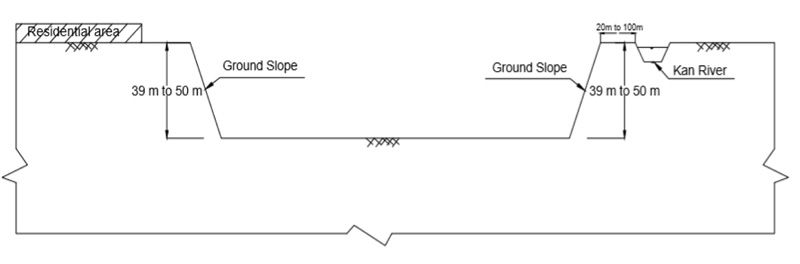) illustrates a section from the deep excavation. A sedimentation process developed the area around the Kan River for thousands of years. The distance between the Kan River and Ferbet excavation on the eastern side is varied from 20 m to 100 m. In order to simplify the model as well as considering the most critical state of the rivers’ position, 20 m distance is assumed in the analyses. The depth of water in the Kan River at the normal condition is about 0.5 m. In the recent intense precipitation, which was rare in Iran, the water depth increased to about 9 m.Fig. (1) The aerial picture of the studied excavation and the neighboring area.Fig. (2) The studied open-pit mine of sand and gravel.Fig. (3) The Kan River, which is located adjacent to the pit (see Fig. 4).

Different layers of soil were identified with the aid of site investigation. According to the site investigation report, the layers were mainly sandy and gravelly. The obtained groundwater-surface from the site investigation report is far from below the excavation. The future architectural plan for the eastern side of the considered excavation is to establish a local recreation center and green space, including to name a few medium-rise buildings, a restaurant, and a retail store,. Additionally, it should be mentioned that only the eastern side of the project is considered for performing reliability and risk analysis in this paper (Fig. 5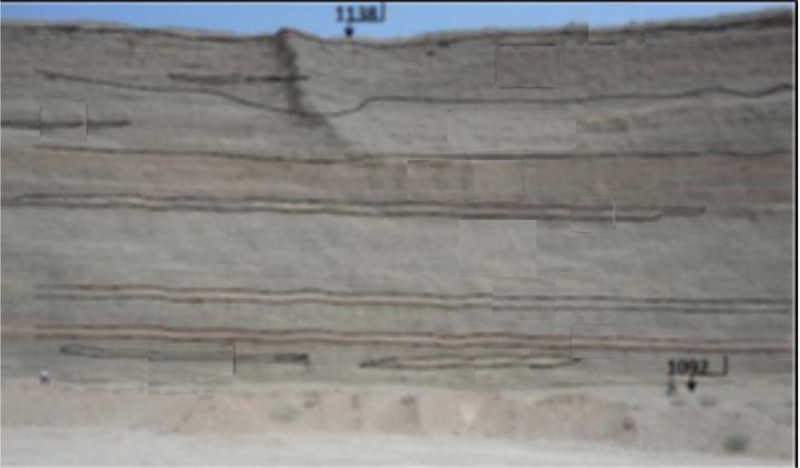). Details on the engineering properties of soils are presented in Table 1.

### 2.2. Slope Stability and Possible Stabilization Methods

#### 2.2.1. Deterministic Stability Analysis of the Basic Excavation

In the first step, the stability analysis is performed using classic, deterministic methods. By numerical modeling of the deep excavation in the basic condition using Plaxis 2D, the factor of safety 1.16 is obtained (Fig. 6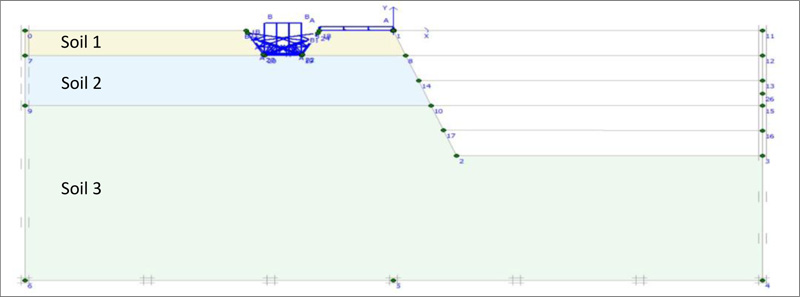). Although it is an acceptable value for safety in temporary conditions, for permanent stability, the factor of safety 1.5 is required. Therefore, the execution of stabilization methods is necessary.Fig. (4) A section of the deep excavations.Fig. (5) The studied wall of the abandoned open pit mine.

Among all possible stabilization methods considering the location of the project, preferences of the owner and future design and purposes of the project, the one with the following features should be opted:

(1) The method should not need various equipment and materials.

(2) Regarding the existing possibilities should be conducted with the lowest cost.

(3) The method should not contradict the plans for the development of green spaces and architectural designs.

In the following, technical and economic assessments are presented to choose an appropriate method.

#### 2.2.2. Technical Assessment of Possible Stabilization Methods

In order to select the best option for the stabilization method, the shortcomings of each one of them with respect to project conditions should be considered. Finally, the best approach should be selected. Gravity walls are restricted to height. Considering the height of excavation, which is about 50 m in some sections, the construction of these retaining walls is not feasible. In addition, the time needed for the construction of some walls such as gravity walls or concrete walls for stabilization of excavations is too long. Although reinforced walls have the possibility of being built in high height, they require special equipment and are difficult to construct. Besides, after the construction of these walls, there is no chance to implement a change in them. According to the fact that the architectural plan of this project is not finalized yet, it is necessary to opt for a more flexible method for future changes. The other proposed method is using sheet piles. This method considering the steel material of sheet piles and weathering and corrosion issues is mostly used in the case of temporary stabilization. Additionally, driving piles in residential areas similar to the problem of interest is not feasible. Another possible method is using nailing or anchors, which are used in both temporary and permanent stabilization. However, in case of permanent stabilization, galvanized covers are needed to control the durability of steel nails and anchors against corrosion. Particularly, some times in the case of anchors, which are pre-tensioned, there is a need to further tension after a while. According to future architectural designs, they may not be easily accessible later. Moreover, providing executive platforms at different levels is essential for the construction process, which is challenging to perform due to excessive length of the wall. Embanking and establishing berm is simple in practice, and there is no need for complicated equipment and professional workforces. The only negative point about this method is providing essential soil materials, which later becomes easy to achieve according to the location of the project. Furthermore, as the project is relatively located far from the center of the city, traffic of the hauling soil truck is easily possible, and this accelerates the construction procedure.

Eventually, according to technical assessment, the embanking system has fewer limitations and is the most proper option technically. In the following, this option is compared to nailing or anchoring systems economically.

### 2.3. Preliminary Economical Assessment

As mentioned earlier, in the problem of interest, using some kind of walls as a stabilization system is not feasible. In Table 2, the embanking system is compared to nailing and anchorage systems economically.

According to Table 2, it is clear that using the embanking method and establishing berm has less cost in comparison to nailing and anchorage systems, due to the simplicity of providing materials and accessibility to materials. Also, this method does not require special equipment for execution.

### 2.4. Selected Stabilization Methods for Further Study Using Reliability Analysis and Risk Assessment

Eventually, considering all the aforementioned reasons, embanking berms were chosen as the appropriate stabilization method in this project. Results for utilization of two kinds of materials for the construction of berms with various depths and shapes were compared. Finally, two shapes were chosen for further investigations.

#### 2.4.1. Berm Stabilization Method (BSM)

It is considered that deep excavation is stable in a temporary condition. However, it is necessary to strengthen it for permanent condition. Therefore, a berm made up of soil with the shape, size, and the properties of space can be constructed as shown in Fig. (7) . The soil parameters of the berm are presented in Table 3.

#### 2.4.2. Cemented Soil Stabilization Method (CSM)

In this option, embanking is implemented by using a mixture of soil and cement, which have higher resistive parameters in comparison to the soil used in BSM. The schematic representation of the proposed CSM is illustrated in Fig. (8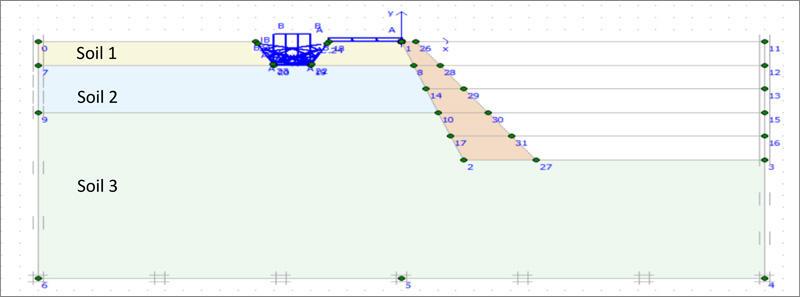). Parameters of cemented soil are presented in Table 4.Fig. (6) Factor of Safety of the critical slope in the basic condition.Fig. (7) The geometry of BSM.Fig. (8) The geometry of CSM.Table 2
Economic comparison of options including nailing, anchorage, and embanking (Berm).

#### 2.4.3. Economic Comparison of BSM and CSM

In order to execute the CSM method, 180 kg cement is needed per cubic meter of soil. Therefore, for 640000 m3 embankment, 115200000 kg cement is required. Considering the unit cost of execution of the CSM method, it costs noticeably higher than the BSM method. Hence, according to Table 5, the BSM method is the optimum method for stabilization of this deep excavation economically.

### 2.5. Reliability Analysis Using Random Set Method and RS-FEM

#### 2.5.1. Random Set Theory

In order to consider the ranges of the parameters that are derived from sensitivity analyses, Random Set Theory will be used.

X can be assumed as an imprecise interval variable, e.g. friction angle of soil. Random set can be defined on X as a pair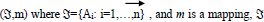[0,1] so that m(Ø)=0, and(1)

In this concept,, contains all the possible values for a variable x and Ai are the central elements, i.e. all possible subsets in the range of X and can be determined as the probability that A is in the range of x. For instance, assumed possible values for the friction angle are between 25 and 40 degrees, and there are three various ranges of friction angle of soil in a specified site, two geotechnical reports and one expert judgment, each of which suggest different values for that. Suppose that the geotechnical report suggested that the friction angle is between 25 to 35. Under this condition, the subset (A1) is between 25 and 35 (Fig. 9). Suppose the second subset (A2) is between 30 and 38, and the third subset (A3) ranged from 32 to 40. Their relative frequency, m, in the above example would be 0.333 for each subset. Total number of a subset (i) varies from i=1 to i=n, where n is the number of available sources [10P.H. Chuang, "Use of fuzzy sets for evaluating shear strength of soils", Comput. Geotech., vol. 17, pp. 425-446.
[http://dx.doi.org/10.1016/0266-352X(95)94914-C]
, 35G.M. Peschl Schweiger, "Reliability analysis in geotechnics with the random set finite element method", Comput. Geotech., vol. 32, no. 6, pp. 422-435.
[http://dx.doi.org/10.1016/j.compgeo.2005.07.002]
, 38H.F. Schweiger, and A. Nasekhian, "A comparison of random set and point estimate methods in finite element analysis of tunnel excavation", Geotechnical Safety and Risk, pp. 341-348.]. Due to the imprecision, only upper and lower bounds of the probability of a complete event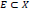can be derived in Random Set theory. In Random Set theory, imprecise probabilities are described by intervals [Bel(E). Pl(E)] that the belief function, Bel, of a subset E, is a set function obtained by way of the summation of basic probability assignments of subsets Ai included in Z; and the plausibility function, Pl, of a subset Z is a set function obtained by means of the summation of basic probability assignments of subsets Ai having non-zero intersection with Z [31F. Tonon, A. Bernardini, and A. Mammino, "Determination of parameters range in rock engineering by means of Random Ret Theory", Reliab. Eng. Syst. Saf., vol. 70, no. 3, pp. 241-261.
[http://dx.doi.org/10.1016/S0951-8320(00)00058-2]
]. The random set theory can be illustrated in a P-box representation system, as shown in Fig. (9) [32F. Tonon, A. Bernardini, and A. Mammino, "Reliability analysis of rock mass response by means of Random Set Theory", Reliab. Eng. Syst. Saf., vol. 70, no. 3, pp. 263-282. [b].
[http://dx.doi.org/10.1016/S0951-8320(00)00059-4]
]. For a restricted interval of a central element, Bel (x) and Pl (x), the lower and upper cumulative probability distribution functions, respectively, can be determined using equations from 2 to 5, at some point x, which are depicted in Fig. (9).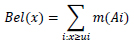(2)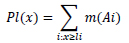(3)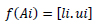(4)

The function ƒ(Ai) illustrates a numerical model in the RS-FEM framework that has to be evaluated 2N times for each central element Ai. The appendix equation suggests the required number of calculations, nc, to specify the bounds of the system.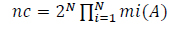(5)

### 2.6. Implementation of RS-FEM for the Selected Stabilization Methods

#### 2.6.1. Modeling Procedure

Peschl [33G.M. Peschl, Reliability analysis in geotechnics with the random set element method, Dissertation, Graz University of Technology, .] proposed RS-FEM initially. Nevertheless, the modeling procedure was summarized by Schweiger et al. [38H.F. Schweiger, and A. Nasekhian, "A comparison of random set and point estimate methods in finite element analysis of tunnel excavation", Geotechnical Safety and Risk, pp. 341-348.] into 7 steps, which are elaborated upon hereunder.

The first step for conducting RS-FEM procedure is defining the geometry of the problem. Finite element model should be prepared, and proper engineering properties of soil layers and support elements should be chosen.

The second step is to identify the influential input parameters on the factor of safety and displacement of the slope as basic variables in RS-FEM as well as determining the ranges of each parameter. As the parameters are reported by different sources of information, including site investigation reports and geotechnical experts, there are two estimations for range of the parameters. The probability of each estimated range is set to 0.5.

The third step is to perform a variance reduction technique to consider spatial variability of soil in random set theory, which requires to estimate the correlation length and the length of the possible failure line.

The fourth step is to operate a sensitivity analysis to reduce the number of input parameters. Since the number of finite element required runs exponentially increases with the number of input variables, it is necessary to identify the most important parameters governing the desirable system response.Fig. (9) Random set bounds construction.

The fifth step is to provide a matrix of different combinations of input parameters. Thereupon, the model outputs for each combination are calculated. A probability is dedicated to the model output for each combination based on the probability of ranges of parameters, which was mentioned before. As an example, the probability of each combination, assuming three basic parameters is 0.5 x 0.5 x 0.5 or 0.125.

The sixth step is to define the lower and upper bounds of the model output based on the results achieved from finite element runs. Afterward, a proper distribution function should be fitted on the bounds using statistical tools.

The seventh step is to opt for a target value and determine the probability of failure based on the fitted distribution function.

#### 2.6.2. Numerical Modeling

Finite element software, PLAXIS 2D, was utilized in order to model the case study. A relatively large working area of 200 m width and 100 m depth was chosen to minimize the boundary effects. The schematic representation was modeled using a plane strain two-dimensional model. The excavation depth is set to be 50 m. A fixed boundary condition was set at the bottom of the model, and the vertical sides of the model were only fixed in the horizontal direction and free in the vertical direction. The soil was modeled using 15-noded elements. A 15-node component can be thought as a composition of four 6-node elements since the total number of nodes and stress points is equal. Nevertheless, one 15-node element is more powerful than four 6-node elements. 15-node element provides a fourth order interpolation for displacements and the numerical integration involves twelve Gauss points (stress points). The 15-node triangle is a very accurate element that can produce high quality stress results for severe issues. Safety factors are generally overpredicted using 6-noded elements. In those cases, the use of 15-node elements is preferred. The total number of elements is 472. Fig. (10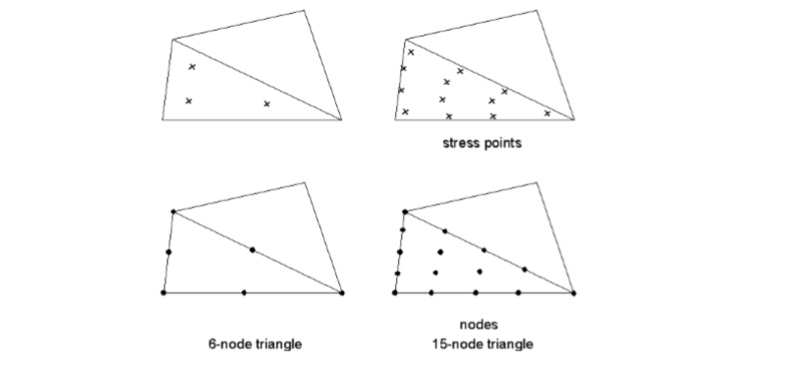) shows the position of nodes and stress points in soil elements. The mesh is shown in Fig. (11).

The soil layers were defined based on the soil profile determined by the geotechnical report. The effect of groundwater was not considered, as its level was far below the bottom of the excavation. Hardening Soil model was chosen as the constitutive model for the soil. Hardening Soil is able to consider stress path and its effect on soil stiffness and soil behavior. Considering the soil profile, the drained behavior of soil was considered. Due to the presence of Kan River in the distance of 20 m to 100 m of the excavation, the water level in the river and the imposed pressure was modeled using the water force on the sides of the embedment of the river. As mentioned before, the water level in Kan River is about 0.5 m in typical condition and about 9 m in intensive precipitation condition.Fig. (10) Position of nodes and stress points in soil elements.Fig. (11) The mesh used for the studied slope.

Stage construction was used for the analysis. In the first stage, initial stresses are generated. Further in steps 2 to 6, the excavation process will be completed. The model schematic representation can be seen in Fig. (6). The main input parameters and material properties used in this study, which are obtained from site investigation and laboratory tests, are presented in Table 1.

#### 2.6.3. Identifying Basic Parameters in the Random Set Model

Determining the basic variables in the RS-FEM model is the primary step in performing finite element analysis [33G.M. Peschl, Reliability analysis in geotechnics with the random set element method, Dissertation, Graz University of Technology, .]. This purpose can be accomplished by sensitivity analysis. However, it is necessary to perform a variance reduction factor in advance. Therefore, in the following description, we elaborate upon the variance reduction factor. Afterward, the explanation about sensitivity analysis will be given.

In the random set theory, parameters are introduced in the form of intervals rather than a constant value. In the cases where mean values of products are presented, the range of the parameters can be determined using the suggested Coefficient of Variances (COV). A recommendation in this regard is presented in Table 6. In order to estimate the upper and lower bounds of the parameters, it is necessary to determine the standard deviation (σ). Equation 6 can be used to determine the standard deviation (σ) for a known mean and COV. The range of the parameter can be determined in terms of mean ± standard deviation. Ranges of parameters used in the considered study are presented in Table 7.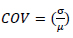(6)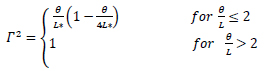(7)(8)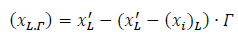(9)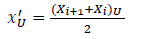(10)(11)

The variance reduction technique is used to consider spatial variability of soil in random set theory. This technique usually leads to a reduction in the amount of uncertainties as a result of adjusting the range of input parameters. The method is proposed by Schweiger and Peschl [35G.M. Peschl Schweiger, "Reliability analysis in geotechnics with the random set finite element method", Comput. Geotech., vol. 32, no. 6, pp. 422-435.
[http://dx.doi.org/10.1016/j.compgeo.2005.07.002]
]. They asserted that assuming n sources of information, the function of the spatial average of the data xi,T can be obtained from the discrete cumulative probability distribution of the field data xi. Where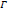is the variance reduction factor, Ɵ is spatial correlation length, and L* is the length of a potential failure surface. Spatial correlation length is suggested to be about 5 to 30 m. For the problem of interest, the correlation length was set to 15. The adjusted ranges of parameters are presented in Table 8. Final results can be easily calculated using spreadsheets. Therefore, only final results are mentioned here.

Sensitivity analysis is used to determine the most influential parameters controlling the desirable system responses. The reduction of the number of basic variables, which leads to a decrease in the number of essential finite element runs, is accomplished using a sensitivity analysis. The method is given by U.S. TRIM [43USEPA T, Total risk integrated methodology status report., US Environmental Protection Agency Office of Air Quality Planning and Standards: Research Triangle Park, .] which was made compatible with the Random set approach by Peschl [33G.M. Peschl, Reliability analysis in geotechnics with the random set element method, Dissertation, Graz University of Technology, .] is used. In this method, three primary coefficients, namely, sensitivity ratio, sensitivity score, and relative sensitivity are considered. The ratio of the change in a model output per unit change of an input variable which is called sensitivity ratio is calculated using Equation 12. In order to accomplish the sensitivity analyses, 4N+1 calculations are needed where N is the number of all parameters.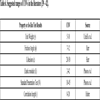Table 6
Suggested ranges of COVs in the literature [39M. Uzielli, S. Lacasse, F. Nadim, and K. Phoon, "Soil variability analysis for geotechnical practice", Proceedings of the second International Workshop on Characterization and Engineering Properties of Natural Soils, .-42M. Huber, Soil variability and its consequences in geotechnical engineering, Inst. f., Geotechnik, .].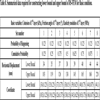Table 8
Summarized data required for constructing lower bound and upper bound of RS-FEM for Basic condition.(12)

Where ηSR is the sensitivity ratio of variable X, XL.R is the upper and lower limits of the local and range intervals of variable X and Xr is the reference value. Sensitivity score can be obtained from the following Equation 13.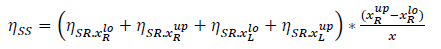(13)

Then the relative sensitivity of the system response A is obtained as follows: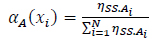(14)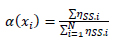(15)

Finally, the total relative sensitivity, α(xi), for each input variable is given by Equation 15.

Respective relative sensitivity should be considered based on the kind of performance function. In this paper, the relative sensitivity of 12 potential parameters for the two aforementioned performance functions was obtained using 49 finite element analysis. Eventually, the most influential parameters were selected by the evaluation of relative sensitivities shown in Fig. (12). Although using the sensitivity score can diminish the effect of input units, using only total sensitivity could be misleading in evaluating the important parameters. It is recommended that influential parameters be selected by taking into account both the total relative sensitivity and the relative sensitivity of an individual system response. According to the values obtained for relative and total sensitivity, the basic parameters for Factor of Safety analysis are Cohesion of first layer, Cohesion of second layer, Cohesion of third layer, and friction angle of third layer. In addition, the basic parameters for displacement analysis are Cohesion, Friction angle, and module of elasticity of third layer. This conclusion does not represent that other parameters are unimportant. Rather, considering many input parameters leads to much more finite element analysis, considering all 12 variables is not possible. For brevity, details of the sensitivity are not presented here and can be found in other studies [33G.M. Peschl, Reliability analysis in geotechnics with the random set element method, Dissertation, Graz University of Technology, ., 38H.F. Schweiger, and A. Nasekhian, "A comparison of random set and point estimate methods in finite element analysis of tunnel excavation", Geotechnical Safety and Risk, pp. 341-348., 44J. Ji, H. Liao, and B.K. Low, "Modeling 2-D spatial variation in slope reliability analysis using interpolated autocorrelations", Comput. Geotech., vol. 40, pp. 135-146.
[http://dx.doi.org/10.1016/j.compgeo.2011.11.002]
].Fig. (12) Relative sensitivities, α (%).

#### 2.6.4. Determination of Lower and Upper Bounds of RS-FEM

The model outputs can construct the upper and lower bounds of the RS-FEM model for Factor of Safety as well as displacement for each combination of basic variables. In this study, the basic variables for Factor of safety are Cohesion of the first layer (C1), Cohesion of the second layer (C2), Cohesion of the third layer (C3) and friction angle of the third layer (F3) and for displacement are Cohesion (C3), friction angle (F3), and Module of elasticity (E3)of third layer. Since parameters are presented as intervals, and there are two sources of information for each parameter, in the case of the factor of safety, there are (24)2 combinations of basic variables, and for displacement, there are (23)2 sets of basic variables. Finite element runs should be conducted for each combination of basic variables in order to determine Factor of safety and displacement for each set. Subsequently, the values for each desired model output should be sorted to construct the upper and lower bounds of the Random set model. The number of basic variables for the Factor of safety and displacement is four and three, respectively. The probability of each value is determined by multiplication of the probability of basic input variables. Therefore, the probability of 0.0625 and 0.125 is assigned to each value of factor of safety and displacement, respectively. This assumption is valid in the case that basic variables are independent of each other. Subsequently, the cumulative probabilities should be determined, which form the Y-axis. The P-box system of response is usually used in the random set theory. For brevity, the sorted values of displacement and Factor of safety, which were obtained in Random set analysis are presented in Tables 8-13 for each stabilization method as well as the basic condition.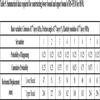Table 9
Summarized data required for constructing lower bound and upper bound of RS-FEM for BSM.Table 10
Summarized data required for constructing lower bound and upper bound of RS-FEM for CSM.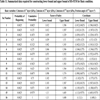Table 11
Summarized data required for constructing lower bound and upper bound of RS-FEM for Basic condition.Table 12
Summarized data required for constructing lower bound and upper bound of RS-FEM for BSM.Table 13
Summarized data required for constructing lower bound and upper bound of RS-FEM for CSM.

#### 2.6.5. Calculation of Probability of Failure

The final step is to fit a distribution function on the bounds of the RS-FEM model and to obtain the probability of failure of the considered case study according to acceptable deformation. To do so, it is necessary to determine the value of deformation for the problem of interest based on literature. In order to compare the calculated values with the acceptable values, determination of the acceptable value of deformation and acceptable probability of failure is necessary. More explanation is provided in the following.

### 2.7. The Use of Performed Reliability Analysis

#### 2.7.1. Acceptable Deformation

The acceptable displacement is determined based on the national codes and engineering judgment. Normalized maximum lateral movement values dh max in clays and sands are frequently between 0.05%H and 0.25%H, where H is the excavation depth [45M. Long, "Database for retaining wall and ground movements due to deep excavations", J. Geotech. Environ. Eng, vol. 127, no. 3, pp. 203-224.
[http://dx.doi.org/10.1061/(ASCE)1090-0241(2001)127:3(203)]
]. Carder [46D. R. Carder, "Ground movements caused by different embedded retaining wall construction techniques", Transport Res.Lab.Rep, vol. 172, Berkshire, U.K, .], based on several reliable case histories in the UK, mentioned that for stiff soils the average value of dh max was 0.2%H. In stiff clays, residual soils and sands, maximum lateral wall movements and settlements of the retained soil average about 0.2% H to 0.3% H [47G.W. Clough, and O.T.D. Rourke, "Construction induced movements of in-situ walls", ]. In cases that there is no important facilities or infrastructure in nearby of the excavation, dh max should be less than 0.7%H [48PSCG, Specification for Excavation in Shanghai Metro Construction., Professional Standards Compilation Group: Shanghai, China, .]. Hence, the acceptable displacement for the problem of the interest is considered 300 mm.

For the stability problem in long-term factor of safety 1.5 is the governing criteria.

#### 2.7.2. Acceptable Probability of Failure

Three types of limit states are considered in reliability analysis; namely, ultimate limit states, serviceability limit states, and fatigue limit states. Ultimate limit states have to do with the loss of load-carrying capacity such as loss of the overall stability. Serviceability limit analysis is related to gradual deterioration, user’s comfort, or maintenance costs, for example, excess deflection and permanent deformations. Fatigue limit states are related to loss of strength under repeated loads [49A. Nowak, and K. Collins, Reliability of Structures, 2nd Edition.]. In the problem of interest, ultimate and serviceability limit states are considered. The acceptable probability of failure (collapse) is reported in a range from 10-4 to 10-6. The probability of serviceability failure due to its less detrimental consequences in comparison to failure of excavation is higher than acceptable probability of failure. As probability of serviceability failure is not mentioned directly in literature, the following method was used to determine the limit state of probability of serviceability.

The reliability index, b, for serviceability limit state is suggested to be 1.5. For the medium consequence of failure, the reliability index is reported at 3.8 [50, 51ISO 13822, Bases for design of structures - Assessment of existing structures, ].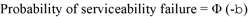(16)

Where Φ indicates the cumulative distribution function.

Nowak and Collins [49A. Nowak, and K. Collins, Reliability of Structures, 2nd Edition.] stated that the probability of serviceability failure could be approximated by the following exponential function.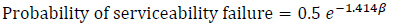(17)

Equation 17 works appropriately as long as the range of reliability index (b) is less than or equal to 2 [52S.H. Ghasemi, Target reliability analysis for structures”, Ph.D. dissertation, Auburn University, ]. By using Equation 17, the probability of serviceability failure for b=1.5 is 0.1 and for b=3.8 is 10-4. Table 14 shows the values for reliability index b and the probability of failure Pf. Overall, the authors suggest the acceptable probability of serviceability equals 0.1.

#### 2.7.3. Calculation of Probability of Excessive Displacement and Probability of Failure

After the determination of the acceptable value of deformation and acceptable probability of failure, the values of probability of excessive displacement and probability of failure are calculated and are presented in Tables 15 and 16, respectively. The acceptable probability of serviceability failure is suggested 0.1 as the target value. The serviceability failure is defined when the horizontal displacement is greater than 300 mm. The probability of serviceability failure is obtained by calculation of the area under the PDF (Probability Density Function) curve. In the presented paper, the probability of serviceability failure is named probability of excessive displacement. In the problem of interest, it was obtained by fitting Gen. Logistic and Pearson distributions on the Random Set outputs.

According to Table 15:

• The probability of excessive displacement for the base case in normal water depth condition (0.5 m) is obtained 0.095, which is not critical. However, in the case of water depth of 9 m, it is 0.126, which is greater than 0.1. Therefore, deep excavation needs to be stabilized.
• The probability of excessive displacement in the BSM for the case of 0.5 m water depth of river for lower and upper bounds is obtained 0 and 0.07195, respectively, and for the case of 9m water depth of river for lower and upper bounds is obtained 0 and 0.07211, respectively. All values mentioned above are less than the acceptable probability of serviceability failure and fulfill the purpose of stabilization.
• The probability of excessive displacement in the CSM for the case of 0.5m water depth of river for lower and upper bounds is obtained 0 and 0.08963, correspondingly, and for the case of 9 m, depth of river for lower and upper bounds is obtained 0 and 0.07195, correspondingly. All values mentioned above are less than the acceptable probability of serviceability failure and fulfill the purpose of stabilization.
• As mentioned before, two possible water depths are considered due to different probable precipitation conditions. The results presented in Table 16 illustrate that although the effect of water depth on the base case is considerable, it has a subtle influence on the probability of excessive displacement after applying the stabilization methods.
• Utilization of stabilization methods (BSM and CSM) leads to a reduction in the probability of excessive displacement of the base case which means that the selected methods act successfully.
• In order to choose the best method for stabilization of the deep excavation, the probability of excessive displacement should be compared in these two cases. It is needless to say that the case with a lower probability of serviceability failure should be opted. For the problem of interest, the BSM has less probability of excessive displacement in comparison to the CSM and is the most efficient method to stabilize deep excavation considered in the present study.

As mentioned before, for the stability problem in long-term, factor of safety 1.5 is the governing criteria. Therefore, the probability of failure is defined when the factor of safety is less than 1.5. The probability of failure is obtained by calculation of the area under the PDF (Probability Density Function) curve. The calculated values are presented in Table 16.

According to Table 16, the probability of failure for BSM is less than this value for CSM. Therefore, as previous results, BSM is the appropriate approach for stabilization of this deep excavation.

### 2.8. Risk Assessment

Risk assessment is defined as the application of a methodology for evaluating risk, where risk is defined as the probability and frequency of occurrence of a hazardous event, exposure of people and property to the hazard and consequences of that exposure [53B. Hu, J. Wang, Z. Chen, D. Wang, and S. Xu, "Risk assessment of land subsidence in Tianjin coastal area in China", Environ. Earth Sci., vol. 59, pp. 269-276.
[http://dx.doi.org/10.1007/s12665-009-0024-6]
]. Hazard is defined as a natural or artificial phenomenon that possesses the potential to disrupt and damage people, property and their immediate environment [54UNISDR, UN International Strategy for Disaster Reduction Sec., .http://www.unisdr.org/we/inform/terminology]. In the presented study, hazard is used to describe the excessive displacement of the slope. Vulnerability can be defined as economic, social and physical factors helping to reduce the ability to deal with the potential hazard impacts. In fact, vulnerability is the characteristic and condition of a system that makes it susceptible to destructions caused by a hazard [54UNISDR, UN International Strategy for Disaster Reduction Sec., .http://www.unisdr.org/we/inform/terminology]. Indeed, the risk is the interaction between hazard and vulnerability.

The level of destruction depends on both the extent of displacement and response of the risk object. Even though a number of structures and utilities can be damaged by excessive displacement, in the presented study, risk objects are limited to buildings and resulting fatality, as well as highways. The economic risk of excessive displacement, Rs, is obtained by a combination of the economic cost, Cs, and the probability of damage, Pf:(18)

First, Rs should be calculated for each risk object. Then, the sum of Rs for all risk objects gives total economic risk for each design alternative [55J. Sundell, E. Haaf, and J. Tornborg, "Comprehensive risk assessment of groundwater drawdown induced subsidence", Stochastic Environ. Res. Risk Assess, vol. 33, pp. 427-449.
[http://dx.doi.org/10.1007/s00477-018-01647-x]
].

### 2.9. Damage Cost Due to Excessive Displacement

In this section, risk analysis is performed. Based on the future architectural plan, some buildings, a highway, and other facilities, which will be mentioned below are considered. In order to assess the risk of various stabilization methods, the approach proposed by HAZUS [56HAZUS MR4, Federal Emergency Management Agency, ] is used. Although HAZUS mainly focuses on earthquake-induced loss estimation, it provides information for risk assessment of other phenomena such as floods and fire. Therefore, the information presented by HAZUS is used in the submitted paper for estimation of loss because of the failure of the slope. HAZUS proposes a classification of various buildings and transportation systems in order to group buildings with similar damage/loss characteristics into a set of predefined building classes and provides the required information for loss estimation. The economic risk calculation is conducted in two sections: 1) Buildings, 2) Highway.

#### 2.9.1. Buildings Damage Cost

A list of considered structures and elaborate descriptions according to HAZUS classification system are presented in Table 17. The considered buildings and their structure type, as well as their area and location, are selected according to the future architectural plan and existing structures.

Firstly, displacements imposed on each structure for all considered cases, including the basic excavation, the BSM, and the CSM were calculated by numerical modeling using Plaxis 2D. Based on the results derived from these analyses and the graph presented by Mair [57R.J. Mair, R.N. Taylor, and J.B. Burland, "Prediction of ground movements and assessment of risk of building damage due to bored tunneling", Proceedings of the International Symposium on Geotechnical Aspects of Underground Construction in Soft Ground, Balkema: LondonRotterdam, pp. 713-718.] damage state of the buildings in both adjacent to the excavation and in the distance about 80 meters of it was obtained.

For each HAZUS occupancy class, a basic default structure full replacement cost (cost per square foot) has been determined. The related values for considered structures are presented in Table 17. It is worth mentioning that the values proposed by HAZUS are acceptable for 2002 in the United States. Therefore, we have converted these values to the present cost (2019) in the location of the problem of the interest, considering the average inflation ratio in the United States and current construction cost in both locations. The final calculated values for the considered excavation location are presented in Table 17. Content replacement value is suggested by HAZUS as a percent of the structure replacement cost. It indicates the percentage of the buildings and their facilities that will be destroyed and should be replaced. Content replacement value is also presented in Table 17. The number of people who are present in the vulnerable zone based on field investigation and expert judgment, and the future architectural plans are presented in Table 17.

HAZUS proposes casualty rates based on building type and damage state. Generally, it defines four types of severity. However, in the presented paper, we focus on the rate of maximum severity, which leads to fatality and does not depict lesser severities of casualties. For the considered severity of casualty, the expected number of occupants killed in a building as a result of the failure in each case is calculated according to equation 14 is presented in Table 18. As mentioned before, the probability of failure in all cases (Basic, BSM, and CSM) is presented in Table 15 eq.(19).

The recent research conducted by Victor Brajer [58V. Brajer, and M. Rahmatian, "From Diye to value of statistical life: A case Study for the Islamic Republic of Iran, International waters learning exchange and resource network", ] estimates a value of statistical life of approximately \$66750, which is about 50 percent greater than our Diye/human capital number. In order to calculate the replacement cost and evaluation of the induced risk, Equation 20 is used.

Calculated values are presented in Table 18. The total risk of the buildings consists of fatality and replacement cost is shown in Table 18.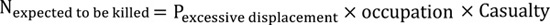(19)(20)

#### 2.9.2. The Highway Damage Cost

The length of the highway adjacent the excavation is 1.2 kilometer. For the highway, damage state was chosen based on the description proposed by HAZUS. HAZUS defines damage state as a term describing the level of damage to each highway system component, namely slight, moderate, extensive, and complete. The numerical modeling output shows several inches of settlement which is categorized in moderate damage state in HAZUS.

As mentioned before, the damage state of the highway is moderate. HAZUS proposes a relationship between the damage state and the damage ratio- indicating the ratio of the repair to the replacement cost for evaluation of economic loss. Accordingly, the damage ratio for roadways with moderate damage state is 0.15 to 0.4. Therefore, it is more sensible to repair the highway rather than replacing it. The repair cost per kilometer of the length of the road after applying the necessary calculations for converting the proposed values to the real values in the location of the excavation in 2019 is shown in Table 19. The total risk of highway damage is presented in Table 20.

## 3. RESULTS AND DISCUSSION

The prioritization of design alternatives can be conducted based on the probability of failure, probability of excessive displacement, or economic risk. For the probability of excessive displacement and failure, the stabilization method with the lowest probability is chosen as the best design alternative. According to the values presented in Table 15, the BSM has the lowest probability of excessive displacement. In the case of probability of failure, the BSM has the lowest value in Table 16 as well. Therefore, based on the comparison of probability of failure and excessive displacement, BSM is the best alternative.

In order to choose the best option according to economic risk, the alternative with the lowest risk should be opted. According to the risk calculation for structures and the highway, the total risk of different proposed methods of stabilization is presented in Table 21. The basic state has the highest risk among all considered cases. On the other hand, BSM has the lowest risk. Therefore, the BSM is appropriate considering the risk analysis.

## CONCLUSION

The purpose of the presented paper is to introduce reliability analysis and risk assessment as techniques, which can be used in decision-making procedure. This article is focused on determining the most appropriate method of stabilization using reliability analysis and risk-based decision-making approach. The following conclusions are obtained.

1- Finite element numerical modeling using Plaxis2D is used to perform deterministic analysis of the excavation. Results showed that Factor of Safety of excavation is not sufficient to be stable in a permanent condition as well as in a probable flood occurrence. In addition, the uncertainty in geotechnical parameters of soil made the deterministic analysis unreliable. Hence, it brings the focus of the authors on probabilistic assessment, reliability analysis, and risk assessment to choose a stabilization system.

2- In order to conduct reliability analysis, RS-FEM, a practical technique in performing reliability analysis, is used. The reliability analysis results are illustrated in a probabilistic form. In the presented paper, the probability of excessive displacement (displacements more than acceptable displacement) and the probability of failure (Factor of Safety less than 1.5) are obtained by fitting the best distribution function on the upper and lower bounds of RS-FEM outputs. Considering calculated probabilities, it is observable that BSM is a more reliable method than CSM, because it has fewer probability values in both of excessive displacement and failure. Based on reliability analysis results, BSM is chosen as the most appropriate method of stabilization in this open-pit mine.

3- In addition to reliability analysis, risk-based decision-making approach is chosen for selecting the most proper stabilization system. In this method, risk is expressed by a combination of the economic cost and the probability of damage for various design alternatives. The procedure includes all destructive events and resulting consequences. Reliability analysis using RS-FEM method can be used for obtaining probability of damage. HAZUS can be successfully adapted to damage cost calculation. Taking into consideration various utilities and infrastructures adjacent to the excavation, the quantified risk of different probable scenarios are calculated and prioritized. According to the risk ranks, BSM has a lower amount of risk in comparison with CSM. Thus, risk assessment results in selecting BSM as the most appropriate stabilization system. The proposed method can be used in similar important projects for the selection of various design alternatives.

Not applicable.

Not applicable.

None.

### CONFLICT OF INTEREST

The authors declare no conflict of interest, financial or otherwise.

Declared none.

## REFERENCES

  S. Mwangi, "Management of river systems in east Africa", Nairobi, Macmillan, .  M. Tariro, Case studies of environmental impacts of sand mining and gravel extraction for urban development in Gaborone, MSc dissertation thesis, University of South Africa, .  N.M. Saviour, "Environmental impacts of soil and sand mining: A Review", Int. J. Sci. Environ. Technol, vol. 1, no. 3, pp. 125-134.  K. Mbaiwa, A Handbook of socio economic and environmental impacts of mining and development, Macmillan: Gaborone, .  R. Schaetzl, "Sand and gravel mining for aggregate", https://www.msu.edu/-soils  A. Nasekhian, and H.F. Schweiger, "Random set finite element method application to tunneling", Int. J. Reliab. Safety, vol. 5, no. 3-4, pp. 299-319.[http://dx.doi.org/10.1504/IJRS.2011.041182]  L.A. Zadeh, "Fuzzy sets", Inf. Control, vol. 8, pp. 338-353.[http://dx.doi.org/10.1016/S0019-9958(65)90241-X]  E. Daniszewska, "Application of the fuzzy set theory to determine the partial factor of safety", Baltic Geodetic Congress (BGC Geomatics), .[http://dx.doi.org/10.1109/BGC.Geomatics.2017.78]  G.R. Dodagoudar, and G. Venkatachalam, "Reliability analysis of slopes using fuzzy sets theory", Comput. Geotech., vol. 27, pp. 101-115.[http://dx.doi.org/10.1016/S0266-352X(00)00009-4]  P.H. Chuang, "Use of fuzzy sets for evaluating shear strength of soils", Comput. Geotech., vol. 17, pp. 425-446.[http://dx.doi.org/10.1016/0266-352X(95)94914-C]  Y. Ben-Haim, and I. Elishakoff, Convex Models of Uncertainty in Applied Mechanics., 1st edElsevier: Amsterdam, .  A.P. Dempster, "Upper and lower probabilities induced by a multivalued mapping", Ann. Math. Stat., pp. 325-339.[http://dx.doi.org/10.1214/aoms/1177698950]  G. Shafer, A mathematical theory of evidence., Princeton University Press: Princeton, .  L.C. Pereira, L.J.N. Guimarães, B. Horowitz, and M. Sánchez, "Coupled hydro-mechanical fault reactivation analysis incorporating evidence theory for uncertainty quantification", Comput. Geotech., vol. 56, pp. 202-215.[http://dx.doi.org/10.1016/j.compgeo.2013.12.007]  D.G. Kendall, Foundations of a theory of random sets., Wiley: New York, .  E. Momeni, M. Poormoosavian, A. Mahdiyar, and A. Fakher, "Evaluating random set technique for reliability analysis of deep urban excavation using Mont Carlo simulation", computers and Geotechnics, vol. 100, pp. 203-215.  M. Ghazian Arabi, and A. Fakher, "Reliability analysis in iran-zamin excavation by application of random set finite element method", The 4th International Reliability Conference, Tabriz, Iran, .  A. Arabani Nezhad, and A. Fakher, "Application of non-probabilistic Random Set method for reliability analysis of deep excavation", The 4th International Reliability Conference, Tabriz, Iran, .  H. Shen, and S.M. Abbas, "Rock slope reliability analysis based on distinct element method and random set theory", Int. J. Rock Mech. Min. Sci., vol. 61, pp. 15-22.[http://dx.doi.org/10.1016/j.ijrmms.2013.02.003]  R.E. Moore, "Interval analysis",  I.R. Goodman, and H.T. Nguyen, ""Uncertainty models for Knowledgebased Systems; A Unified Approach to the Measurement of Uncertainty"",  H. Yang, Y. Li, and Y. Xue, "Interval uncertainty analysis of elastic bi-modular truss structures", Inverse Probl. Sci. Eng., vol. 23, pp. 578-589.  D. Chen, D. Xu, G. Ren, Q. Jiang, G. Liu, L. Wan, and N. Li, "Simulation of cross-correlated non-Gaussian random fields for layered rock mass mechanical parameters", Comput. Geotech., vol. 112, pp. 104-119.[http://dx.doi.org/10.1016/j.compgeo.2019.04.012]  A.P. Dyson, and A. Tolooiyan, "Prediction and classification for finite element slope stability analysis by random field comparison", Comput. Geotech., vol. 109, pp. 117-129.[http://dx.doi.org/10.1016/j.compgeo.2019.01.026]  M.S. Masoudian, M.A. Hashemi Afrapoli, A. Tasalloti, and A.M. Marshall, A general framework for coupled hydro-mechanical modelling of rainfall-induced instability in unsaturated slopes with multivariate random fields., vol. Vol. 115, Computers and Geotechnics, .  L. Liu, Y. Cheng, and S. Zhang, "Conditional random field reliability analysis of a cohesion-frictional slope", Comput. Geotech., vol. 82, pp. 173-186.[http://dx.doi.org/10.1016/j.compgeo.2016.10.014]  Y.J. Li, M.A. Hicks, and P.J. Vardon, "Uncertainty reduction and sampling efficiency in slope designs using 3D conditional random fields", Comput. Geotech., vol. 79, pp. 159-172.[http://dx.doi.org/10.1016/j.compgeo.2016.05.027]  J. Rohmer, and C. Baudrit, "The use of the possibility theory to investigate the epistemic uncertainties within scenario-based earthquake risk assessments", Nat. Hazards, vol. 56, pp. 613-632.[http://dx.doi.org/10.1007/s11069-010-9578-6]  P. Walley, Statistical reasoning with imprecise probabilities., Chapman and Hall: London, .[http://dx.doi.org/10.1007/978-1-4899-3472-7]  F. Tonon, A. Mammino, and A. Bernardini, "A random set approach to the uncertainties in rock engineering and tunnel lining design", Proceedings of ISRM International Symposium on Prediction and Performance in Rock Mechanics and Rock Engineering (EUROCK ’96, vol. 2, pp. 861-868.  F. Tonon, A. Bernardini, and A. Mammino, "Determination of parameters range in rock engineering by means of Random Ret Theory", Reliab. Eng. Syst. Saf., vol. 70, no. 3, pp. 241-261.[http://dx.doi.org/10.1016/S0951-8320(00)00058-2]  F. Tonon, A. Bernardini, and A. Mammino, "Reliability analysis of rock mass response by means of Random Set Theory", Reliab. Eng. Syst. Saf., vol. 70, no. 3, pp. 263-282. [b].[http://dx.doi.org/10.1016/S0951-8320(00)00059-4]  G.M. Peschl, Reliability analysis in geotechnics with the random set element method, Dissertation, Graz University of Technology, .  H.F. Schweiger, and G.M. Peschl, "Basic concepts and applications of random sets in geotechnical engineering", Book Series CISM International Centre for Mechanical Sciences, vol. 491, pp. 113-126.[http://dx.doi.org/10.1007/978-3-211-73366-0_4]  G.M. Peschl Schweiger, "Reliability analysis in geotechnics with the random set finite element method", Comput. Geotech., vol. 32, no. 6, pp. 422-435.[http://dx.doi.org/10.1016/j.compgeo.2005.07.002]  R. T., Clemen, Making Hard Decision., 2nd edDuxbury Press: United States of America, .  W.K. Kong, "Risk assessment of slopes", Q. J. Eng. Geol. Hydrogeol., vol. 35, no. 3, pp. 213-222.[http://dx.doi.org/10.1144/1470-9236/2000-39]  H.F. Schweiger, and A. Nasekhian, "A comparison of random set and point estimate methods in finite element analysis of tunnel excavation", Geotechnical Safety and Risk, pp. 341-348.  M. Uzielli, S. Lacasse, F. Nadim, and K. Phoon, "Soil variability analysis for geotechnical practice", Proceedings of the second International Workshop on Characterization and Engineering Properties of Natural Soils, .  M.E. Harr, Reliability-based design in civil engineering., McGraw-Hill: New York, .  K-K. Phoon, and K.H. Kulhawy, "Characterization of geotechnical variability", Can. Geotech. J., vol. 36, no. 4, pp. 612-624.[http://dx.doi.org/10.1139/t99-038]  M. Huber, Soil variability and its consequences in geotechnical engineering, Inst. f., Geotechnik, .  USEPA T, Total risk integrated methodology status report., US Environmental Protection Agency Office of Air Quality Planning and Standards: Research Triangle Park, .  J. Ji, H. Liao, and B.K. Low, "Modeling 2-D spatial variation in slope reliability analysis using interpolated autocorrelations", Comput. Geotech., vol. 40, pp. 135-146.[http://dx.doi.org/10.1016/j.compgeo.2011.11.002]  M. Long, "Database for retaining wall and ground movements due to deep excavations", J. Geotech. Environ. Eng, vol. 127, no. 3, pp. 203-224.[http://dx.doi.org/10.1061/(ASCE)1090-0241(2001)127:3(203)]  D. R. Carder, "Ground movements caused by different embedded retaining wall construction techniques", Transport Res.Lab.Rep, vol. 172, Berkshire, U.K, .  G.W. Clough, and O.T.D. Rourke, "Construction induced movements of in-situ walls",  PSCG, Specification for Excavation in Shanghai Metro Construction., Professional Standards Compilation Group: Shanghai, China, .  A. Nowak, and K. Collins, Reliability of Structures, 2nd Edition.  ISO 2394, General principles on reliability for structures,  ISO 13822, Bases for design of structures - Assessment of existing structures,  S.H. Ghasemi, Target reliability analysis for structures”, Ph.D. dissertation, Auburn University,  B. Hu, J. Wang, Z. Chen, D. Wang, and S. Xu, "Risk assessment of land subsidence in Tianjin coastal area in China", Environ. Earth Sci., vol. 59, pp. 269-276.[http://dx.doi.org/10.1007/s12665-009-0024-6]  UNISDR, UN International Strategy for Disaster Reduction Sec., .http://www.unisdr.org/we/inform/terminology  J. Sundell, E. Haaf, and J. Tornborg, "Comprehensive risk assessment of groundwater drawdown induced subsidence", Stochastic Environ. Res. Risk Assess, vol. 33, pp. 427-449.[http://dx.doi.org/10.1007/s00477-018-01647-x]  HAZUS MR4, Federal Emergency Management Agency,  R.J. Mair, R.N. Taylor, and J.B. Burland, "Prediction of ground movements and assessment of risk of building damage due to bored tunneling", Proceedings of the International Symposium on Geotechnical Aspects of Underground Construction in Soft Ground, Balkema: LondonRotterdam, pp. 713-718.  V. Brajer, and M. Rahmatian, "From Diye to value of statistical life: A case Study for the Islamic Republic of Iran, International waters learning exchange and resource network",

### Endorsements

"We greatly appreciate the efficient, professional and rapid processing of our paper by your team. The editors are so kind and professional to send us the reviewers' feedback in time. Those comments were all valuable and very helpful for us in revising and improving our paper."

Hailong Zhao
School of Civil Engineering,
Tianjin University, Tianjin,
China

Webmaster Contact: info@benthamopen.net